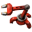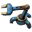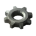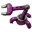## Evaluation order of circuit entities

Don't know how to use a machine? Looking for efficient setups? Stuck in a mission?
FunMaker
Long Handed InserterPosts: 72
Joined: Wed Jun 08, 2016 8:43 pm
Contact:

### Evaluation order of circuit entities

I searched the forum and internet and did not find any proper answer. I darkly remember, that the evaluation order of circiut entities in the order of placement in the world. Is this correct?

The question for me is:
If i have a clock for synchronization of all calculations of other circuit entities.
It counts to 60 and resets to 0 and repeats . Every time at 30 it pulses a "clock value" to the other entities:
Will all combinators evaluate this clock in the same frame?

Or will it be like:
Frame A
• Entity X reads clock value = 0
• clock sets clock value = 1
• Entity Y reads clock value = 1
Frame A + 1
• Entity X reads clock value = 1
• clock sets clock value = 0
• Entity X reads clock value = 0
Depending on the placement of the entities?

MassiveDynamic
Fast InserterPosts: 146
Joined: Sun Sep 16, 2018 10:44 pm
Contact:

### Re: Evaluation order of circuit entities

Each combinator adds one game tick to the signal. But circuit wires connected to electric poles all carry the same signal simultaneously.
So as long as all of your inputs are wired from output to electric poles to next input all calculations at this level will happen in the same tick.

I think that should answer your question as I understand it. Although my lingo may be somewhat pedestrian.

FunMaker
Long Handed InserterPosts: 72
Joined: Wed Jun 08, 2016 8:43 pm
Contact:

### Re: Evaluation order of circuit entities

Not exactly.

My question is:
Will all entities see a change of value in the same tick?
Imagine a network:
- One Combinator outputs nothing in tick 0, A = 1 in tick 1, and A = 2 in tick 2
- Other combinators read in tick 0, tick 1, tick 2
There are 2 possibilities:
Option 1:
Entities read different values in each tick:
Some Entities: 0,0,1,(2 in tick 3)
Other Entities: 0,1,2
Option 2:
All entities will read the same value:0,1,2

This depends on how the game engine is made. If the trigger to update the network is "Value is changed", then it would be Option 2.
If it is "Iterate through all entities in the network" it will be Option 1 because the entity, that outputs the value might be evaluated (by the game engine) later than other entities that will read the value, so these entities will read the value before it is updated.

Koub
Global ModeratorPosts: 6029
Joined: Fri May 30, 2014 8:54 am
Contact:

### Re: Evaluation order of circuit entities

[Koub] Moved to Gameplay Help.
Koub - Please consider English is not my native language.

mmmPI
Filter InserterPosts: 533
Joined: Mon Jun 20, 2016 6:10 pm
Contact:

### Re: Evaluation order of circuit entities

You can test any of the 2 behavior in game by using the editor, (/editor) it has a function that allows you to proceed tick by tick.

I am not sure i understand what would be the effect in game though. And if there is no difference in game i can't understand why it would matter.

I think none of option 1 or 2 is correct . Every tick, every thing is processed. Also each operation need 1 tick to perform.

If you have a clock that goes 1 2 3 4 5 6 and link that to a power pole to read it:

then tick 1 you see 1 tick 2 you see 2 tick 3 you see 3.

an arithmetic combinator taking this as an input and doing +1 will act this way (i think):

tick 1 receive 1, output 1 ( 0+1)
tick 2 receive 2, output 2 (1+1)
tick 3 receive 3, output 3 (2+1)

each tick the input is updated, and each tick the ouput is the result of the previous input plus the operation you perfomed on it.
hence at tick 2, you don't read 3, only the input is 2, the operation has not been performed yet on the input(2+1) it has just received, instead it ouput the result of the previous tick operation(1+1).

If you were to have a clock that goes 1 2 3 4 5 6
and you link in serie 6 arithmetic combinator that does +0 you would read

Tick 1: clock 1, 1rst arithmetic input 1 output 0; 2n darithmetic input 0 ouput 0 ; 3a, i =0 , o=0 ; 4a i=0, o=0 ; 5a i=0 , o=0 : 6a i=0 , o=0.

Tick 2 : C=2, 1a i=2, o=1; 2a i=1 o=0 ; 3a, i =0 , o=0 ; 4a i=0, o=0 ; 5a i=0 , o=0 : 6a i=0 , o=0.
tick 3 : C=3, 1a i=3, o=2; 2a i=2 o=1 ; 3a, i =1 , o=0 ; 4a i=0, o=0 ; 5a i=0 , o=0 : 6a i=0 , o=0.
tick 4 : C=4, 1a i=4, o=3; 2a i=3 o=2 ; 3a, i =2 , o=1 ; 4a i=1, o=0 ; 5a i=0 , o=0 : 6a i=0 , o=0.
tick 5 : C=5, 1a i=5, o=4; 2a i=4 o=3 ; 3a, i =3 , o=2 ; 4a i=2, o=1 ; 5a i=1 , o=0 : 6a i=0 , o=0.
tick 6 : C=6, 1a i=6, o=5; 2a i=5 o=4 ; 3a, i =4 , o=3 ; 4a i=3, o=2 ; 5a i=2 , o=1 : 6a i=1 , o=0.
tick 7 : C=7, 1a i=7, o=6; 2a i=6 o=5 ; 3a, i =5 , o=4 ; 4a i=4, o=3 ; 5a i=3 , o=2 : 6a i=2 , o=1.

I expect the 6a ( 6th arithmetic of the serie) to show the signal from the clock with a 6 tick delay, the time the signal propagate.

between tick 1 and tick 7 there are 6 updates, hence the result is visible only at tick 7.

The ouput of a combinator is equal to the input of the next one at any time.
The output of a combinator is updated each tick, based on the value of the input from the previous tick.
Last edited by mmmPI on Sun Oct 04, 2020 9:52 pm, edited 1 time in total.

Khagan
Long Handed InserterPosts: 60
Joined: Mon Mar 25, 2019 9:40 pm
Contact:

### Re: Evaluation order of circuit entities

FunMaker wrote:
Sun Oct 04, 2020 9:23 pm
My question is:
Will all entities see a change of value in the same tick?
The same tick as each other, yes. The change of value is seen by all entities the tick after it is calculated.

FunMaker
Long Handed InserterPosts: 72
Joined: Wed Jun 08, 2016 8:43 pm
Contact:

### Re: Evaluation order of circuit entities

mmmPI wrote:
Sun Oct 04, 2020 9:47 pm
You can test any of the 2 behavior in game by using the editor, (/editor) it has a function that allows you to proceed tick by tick.

I am not sure i understand what would be the effect in game though. And if there is no difference in game i can't understand why it would matter.

I think none of option 1 or 2 is correct . Every tick, every thing is processed. Also each operation need 1 tick to perform.

If you have a clock that goes 1 2 3 4 5 6 and link that to a power pole to read it:

then tick 1 you see 1 tick 2 you see 2 tick 3 you see 3.

an arithmetic combinator taking this as an input and doing +1 will act this way (i think):

tick 1 receive 1, output 1 ( 0+1)
tick 2 receive 2, output 2 (1+1)
tick 3 receive 3, output 3 (2+1)

each tick the input is updated, and each tick the ouput is the result of the previous input plus the operation you perfomed on it.
hence at tick 2, you don't read 3, only the input is 2, the operation has not been performed yet on the input(2+1) it has just received, instead it ouput the result of the previous tick operation(1+1).

If you were to have a clock that goes 1 2 3 4 5 6
and you link in serie 6 arithmetic combinator that does +0 you would read

Tick 1: clock 1, 1rst arithmetic input 1 output 0; 2n darithmetic input 0 ouput 0 ; 3a, i =0 , o=0 ; 4a i=0, o=0 ; 5a i=0 , o=0 : 6a i=0 , o=0.

Tick 2 : C=2, 1a i=2, o=1; 2a i=1 o=0 ; 3a, i =0 , o=0 ; 4a i=0, o=0 ; 5a i=0 , o=0 : 6a i=0 , o=0.
tick 3 : C=3, 1a i=3, o=2; 2a i=2 o=1 ; 3a, i =1 , o=0 ; 4a i=0, o=0 ; 5a i=0 , o=0 : 6a i=0 , o=0.
tick 4 : C=4, 1a i=4, o=3; 2a i=3 o=2 ; 3a, i =2 , o=1 ; 4a i=1, o=0 ; 5a i=0 , o=0 : 6a i=0 , o=0.
tick 5 : C=5, 1a i=5, o=4; 2a i=4 o=3 ; 3a, i =3 , o=2 ; 4a i=2, o=1 ; 5a i=1 , o=0 : 6a i=0 , o=0.
tick 6 : C=6, 1a i=6, o=5; 2a i=5 o=4 ; 3a, i =4 , o=3 ; 4a i=3, o=2 ; 5a i=2 , o=1 : 6a i=1 , o=0.
tick 7 : C=7, 1a i=7, o=6; 2a i=6 o=5 ; 3a, i =5 , o=4 ; 4a i=4, o=3 ; 5a i=3 , o=2 : 6a i=2 , o=1.

I expect the 6a ( 6th arithmetic of the serie) to show the signal from the clock with a 6 tick delay, the time the signal propagate.

between tick 1 and tick 7 there are 6 updates, hence the result is visible only at tick 7.

The ouput of a combinator is equal to the input of the next one at any time.
The output of a combinator is updated each tick, based on the value of the input from the previous tick.
Khagan wrote:
Sun Oct 04, 2020 9:52 pm
FunMaker wrote:
Sun Oct 04, 2020 9:23 pm
My question is:
Will all entities see a change of value in the same tick?
The same tick as each other, yes. The change of value is seen by all entities the tick after it is calculated.
Okay. That were the answer that i looked for. Thanks! So the value is changed and not published to the other entities before the next tick begins. And the order of evaluation by the game engine does not matter.

mergele
Fast InserterPosts: 142
Joined: Sat Aug 20, 2016 5:45 am
Contact:

### Re: Evaluation order of circuit entities

Yes everyone works on the same set of inputs, when everyone is finished the results are added up and pushed onto the circuit as new input for the next round of operations.

### Who is online

Users browsing this forum: No registered users